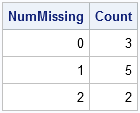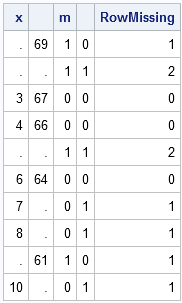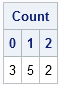This is Part 4 of my response to Charlie Huang's interesting article titled Top 10 most powerful functions for PROC SQL. As I did for eaerlier topics, I will examine one of the "powerful" SQL functions that Charlie mentions and show how to do the same computation in SAS/IML software. Today's topic: finding (and counting) missing values.

### Detecting and Counting Missing Values in PROC SQL

Charlie uses the MISSING function in PROC SQL to detect missing values in observations. The example used in his post is similar to the following:

```data B; input x1 x2 @@; datalines; . 69 . . 3 67 4 66 . . 6 64 7 . 8 . . 61 10 . ;   proc sql ; title 'Missing() -- Return Boolean value for any missing obs'; select sum(missing(x1), missing(x2)) as NumMissing, count(calculated NumMissing) as Count from B group by calculated NumMissing; quit;```### Detecting Missing Values in PROC IML

The MISSING function is part of Base SAS software and you can call Base SAS function from PROC IML, so you can use the MISSING function to detect missing values in PROC IML, as follows:

```proc iml; use B; read all var {x1 x2} into x; close B; m = missing(x); /** matrix of 0s and 1s **/ RowMissing = m[,+]; /** sum across columns **/ print x m RowMissing;```In fact, there are many options for detecting missing values in PROC IML. Given a matrix x, each of the following methods creates a matrix of zeros and ones that is the same dimension as x. The zeros correspond to nonmissing elements; the ones to missing elements.

• Use the NMISS function to detect numerical missing values.
• In SAS 9.2, use the CMISS function to detect missing values in either numerical or character variables.
• Use the notation m = (x=.); to detect numerical missing values.
• Use the notation m = (x=""); to detect character missing values.

After you detect the missing values, you can remove the rows that have one or more missing values.

### Counting Missing Values in PROC IML

Although there are several ways to detect missing values, my favorite way to count the number of missing values in each row is to use the UNIQUE/LOC technique, which is described on p. 69 of my book, Statistical Programming with SAS/IML Software. The following example, counts the number of rows that have zero, one, or two missing values:

```/** use UNIQUE/LOC technique to count missing **/ u = unique(RowMissing); Count = j(1, ncol(u)); do i = 1 to ncol(u); Count[i] = sum(RowMissing = u[i]); end; labels = putn(u, "BEST3."); print Count[colname=labels];```Share

### About AuthorDistinguished Researcher in Computational Statistics

Rick Wicklin, PhD, is a distinguished researcher in computational statistics at SAS and is a principal developer of PROC IML and SAS/IML Studio. His areas of expertise include computational statistics, simulation, statistical graphics, and modern methods in statistical data analysis. Rick is author of the books Statistical Programming with SAS/IML Software and Simulating Data with SAS.